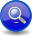#Boost C++ Libraries

...one of the most highly regarded and expertly designed C++ library projects in the world.

##### Repetition Parser Directive (`repeat[]`)
###### Description

The `repeat[]` provides a more powerful and flexible mechanism for repeating a parser. There are grammars that are impractical and cumbersome, if not impossible, for the basic EBNF iteration syntax (Kleene and the Plus) to specify. Examples:

• A file name may have a maximum of 255 characters only.
• A specific bitmap file format has exactly 4096 RGB color information.
• A 256 bit binary string (1..256 1s or 0s).
###### Header
```// forwards to <boost/spirit/home/qi/directive/repeat.hpp>
#include <boost/spirit/include/qi_repeat.hpp>
```

Also, see Include Structure.

###### Namespace

Name

```boost::spirit::repeat // alias: boost::spirit::qi::repeat```

```boost::spirit::inf // alias: boost::spirit::qi::inf```

###### Model of

Notation

`a`
`n`, `min`, `max`

An `int` anything that can be converted to an `int`, or a Lazy Argument that evaluates to anything that can be converted to an `int`.

###### Expression Semantics

Semantics of an expression is defined only where it differs from, or is not defined in `UnaryParser`.

Expression

Semantics

`repeat[a]`

Repeat `a` zero or more times. Same as Kleene.

`repeat(n)[a]`

Repeat `a` exactly `n` times.

```repeat(min, max)[a]```

Repeat `a` at least `min` times and at most `max` times.

```repeat(min, inf)[a]```

Repeat `a` at least `min` or more (continuing until `a` fails or the input is consumed).

###### Attributes

Expression

Attribute

`repeat[a]`

```a: A --> repeat[a]: vector<A>
a: Unused --> repeat[a]: Unused```

`repeat(n)[a]`

```a: A --> repeat(n)[a]: vector<A>
a: Unused --> repeat(n)[a]: Unused```

```repeat(min, max)[a]```

```a: A --> repeat(min, max)[a]: vector<A>
a: Unused --> repeat(min, max)[a]: Unused```

```repeat(min, inf)[a]```

```a: A --> repeat(min, inf)[a]: vector<A>
a: Unused --> repeat(min, inf)[a]: Unused```

###### Complexity

The overall complexity is defined by the complexity of its subject parser. The complexity of `repeat` itself is O(N), where N is the number of repetitions to execute.

###### Example
NoteThe test harness for the example(s) below is presented in the Basics Examples section.

Using the repeat directive, we can now write our examples above.

Some using declarations:

```using boost::spirit::qi::repeat;
using boost::spirit::qi::lit;
using boost::spirit::qi::uint_parser;
using boost::spirit::qi::_1;
using boost::spirit::ascii::char_;
namespace phx = boost::phoenix;
```

A parser for a file name with a maximum of 255 characters:

```test_parser("batman.jpeg", repeat(1, 255)[char_("a-zA-Z_./")]);
```

A parser for a specific bitmap file format which has exactly 4096 RGB color information. (for the purpose of this example, we will be testing only 3 RGB color information.)

```uint_parser<unsigned, 16, 6, 6> rgb;
std::vector<unsigned> colors;
test_parser_attr("ffffff0000003f3f3f", repeat(3)[rgb], colors);
std::cout
<< std::hex
<< colors << ','
<< colors << ','
<< colors << std::endl;
```

A 256 bit binary string (1..256 1s or 0s). (For the purpose of this example, we will be testing only 16 bits.)

```test_parser("1011101011110010", repeat(16)[lit('1') | '0']);
```

The Loop parsers can be dynamic. Consider the parsing of a binary file of Pascal-style length prefixed string, where the first byte determines the length of the incoming string. Here's a sample input:This trivial example cannot be practically defined in traditional EBNF. Although some EBNF variants allow more powerful repetition constructs other than the Kleene Star, we are still limited to parsing fixed strings. The nature of EBNF forces the repetition factor to be a constant. On the other hand, Spirit allows the repetition factor to be variable at run time. We could write a grammar that accepts the input string above. Example using phoenix:

```std::string str;
int n;
test_parser_attr("\x0bHello World",
char_[phx::ref(n) = _1] >> repeat(phx::ref(n))[char_], str);
std::cout << n << ',' << str << std::endl;  // will print "11,Hello World"
```

 Copyright © 2001-2011 Joel de Guzman, Hartmut Kaiser Distributed under the Boost Software License, Version 1.0. (See accompanying file LICENSE_1_0.txt or copy at http://www.boost.org/LICENSE_1_0.txt)